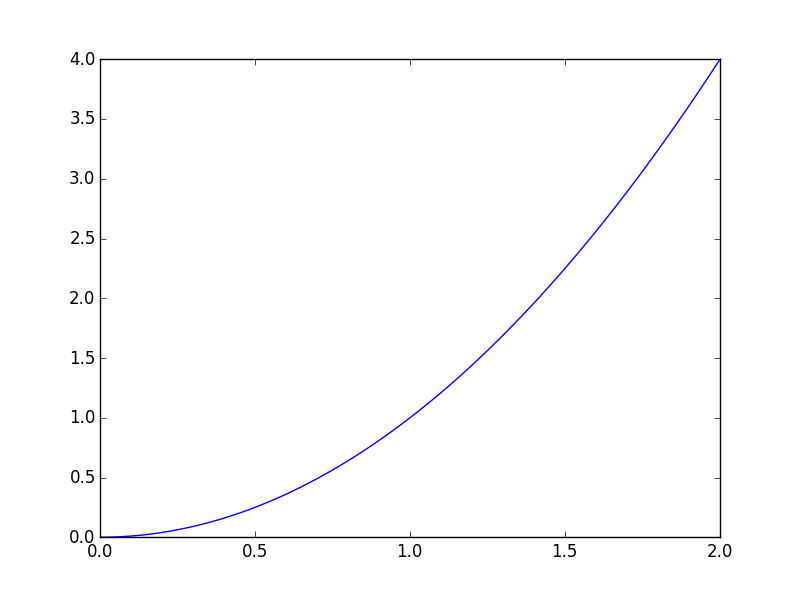# [Solved] How to plot a math function from string?

You can turn a string into code by using pythons `eval` function, but this is dangerous and generally considered bad style, See this: https://stackoverflow.com/a/661128/3838691.
If user can input the string, they could input something like
`import subprocess; subprocess.check_call(['rm', '-rf', '*'], shell=True)`.

So be sure that you build in reasonable security into this.

You can define a function that takes a string and returns a function.
We need to do a little preprocessing to allow the user to input formulas more like he is used to (^ etc.):

## Edit: Second version – white list instead of blacklist

It seems better to define allowed and supported words than blacklisting some:

``````import re

replacements = {
'sin' : 'np.sin',
'cos' : 'np.cos',
'exp': 'np.exp',
'sqrt': 'np.sqrt',
'^': '**',
}

allowed_words = [
'x',
'sin',
'cos',
'sqrt',
'exp',
]

def string2func(string):
''' evaluates the string and returns a function of x '''
# find all words and check if all are allowed:
for word in re.findall('[a-zA-Z_]+', string):
if word not in allowed_words:
raise ValueError(
'"{}" is forbidden to use in math expression'.format(word)
)

for old, new in replacements.items():
string = string.replace(old, new)

def func(x):
return eval(string)

return func

if __name__ == '__main__':

func = string2func(input('enter function: f(x) = '))
a = float(input('enter lower limit: '))
b = float(input('enter upper limit: '))
x = np.linspace(a, b, 250)

plt.plot(x, func(x))
plt.xlim(a, b)
plt.show()
``````

Result:

``````\$ python test.py
enter function: f(x) = x^2
enter lower limit: 0
enter upper limit: 2
``````And for a malicious user:

``````enter function: f(x) = import subprocess; subprocess.check_call(['rm', '-rf', '*'], shell=True)
Traceback (most recent call last):
File "test.py", line 35, in <module>
func = string2func(input('enter function: f(x) = '))
File "test.py", line 22, in string2func
'"{}" is forbidden to use in math expression'.format(word)
ValueError: "import" is forbidden to use in math expression
``````

## Edit: First version – blacklist hazardous words:

``````import numpy as np
import matplotlib.pyplot as plt

# there should be a better way using regex
replacements = {
'sin' : 'np.sin',
'cos' : 'np.cos',
'exp': 'np.exp',
'^': '**',
}

# think of more security hazards here
forbidden_words = [
'import',
'shutil',
'sys',
'subprocess',
]

def string2func(string):
''' evaluates the string and returns a function of x '''
for word in forbidden_words:
if word in string:
raise ValueError(
'"{}" is forbidden to use in math expression'.format(word)
)

for old, new in replacements.items():
string = string.replace(old, new)

def func(x):
return eval(string)

return func
``````

solved How to plot a math function from string?